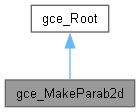# gce_MakeParab2d Class Reference

This class implements the following algorithms used to create Parab2d from gp. Defines an infinite parabola. An axis placement one axis defines the local cartesian coordinate system ("XAxis") of the parabola. The vertex of the parabola is the "Location" point of the local coordinate system of the parabola. The "XAxis" of the parabola is its axis of symmetry. The "XAxis" is oriented from the vertex of the parabola to the Focus of the parabola. The "YAxis" is parallel to the directrix of the parabola and its "Location" point is the vertex of the parabola. The equation of the parabola in the local coordinate system is Y**2 = (2*P) * X P is the distance between the focus and the directrix of the parabola called Parameter). The focal length F = P/2 is the distance between the vertex and the focus of the parabola. More...

`#include <gce_MakeParab2d.hxx>`

Inheritance diagram for gce_MakeParab2d:[legend]

## Public Member Functions

gce_MakeParab2d (const gp_Ax2d &MirrorAxis, const Standard_Real Focal, const Standard_Boolean Sense=Standard_True)
Creates a parabola with its axis of symmetry ("MirrorAxis") and its focal length. Warnings : It is possible to have Focal = 0. The status is "NullFocalLength" Raised if Focal < 0.0. More...

gce_MakeParab2d (const gp_Ax22d &A, const Standard_Real Focal)
Creates a parabola with its local coordinate system and its focal length. Warnings : It is possible to have Focal = 0. The status is "NullFocalLength" Raised if Focal < 0.0. More...

gce_MakeParab2d (const gp_Ax2d &D, const gp_Pnt2d &F, const Standard_Boolean Sense=Standard_True)
Creates a parabola with the directrix and the focus point. The sense of parametrization is given by Sense. More...

gce_MakeParab2d (const gp_Pnt2d &S1, const gp_Pnt2d &Center, const Standard_Boolean Sense=Standard_True)
Make an Parab2d with S1 as the Focal point and Center as the apex of the parabola Warning The MakeParab2d class does not prevent the construction of a parabola with a null focal distance. If an error occurs (that is, when IsDone returns false), the Status function returns: More...

const gp_Parab2dValue () const
Returns the constructed parabola. Exceptions StdFail_NotDone if no parabola is constructed. More...

const gp_Parab2dOperator () const

operator gp_Parab2d () constPublic Member Functions inherited from gce_Root
Standard_Boolean IsDone () const
Returns true if the construction is successful. More...

gce_ErrorType Status () const
Returns the status of the construction: More...Protected Attributes inherited from gce_Root
gce_ErrorType TheError

## Detailed Description

This class implements the following algorithms used to create Parab2d from gp. Defines an infinite parabola. An axis placement one axis defines the local cartesian coordinate system ("XAxis") of the parabola. The vertex of the parabola is the "Location" point of the local coordinate system of the parabola. The "XAxis" of the parabola is its axis of symmetry. The "XAxis" is oriented from the vertex of the parabola to the Focus of the parabola. The "YAxis" is parallel to the directrix of the parabola and its "Location" point is the vertex of the parabola. The equation of the parabola in the local coordinate system is Y**2 = (2*P) * X P is the distance between the focus and the directrix of the parabola called Parameter). The focal length F = P/2 is the distance between the vertex and the focus of the parabola.

• Create a Parab2d from one apex and the center.
• Create a Parab2d with the directrix and the focus point.
• Create a Parab2d with its vertex point and its axis of symmetry and its focus length.

## ◆ gce_MakeParab2d() [1/4]

 gce_MakeParab2d::gce_MakeParab2d ( const gp_Ax2d & MirrorAxis, const Standard_Real Focal, const Standard_Boolean Sense = `Standard_True` )

Creates a parabola with its axis of symmetry ("MirrorAxis") and its focal length. Warnings : It is possible to have Focal = 0. The status is "NullFocalLength" Raised if Focal < 0.0.

## ◆ gce_MakeParab2d() [2/4]

 gce_MakeParab2d::gce_MakeParab2d ( const gp_Ax22d & A, const Standard_Real Focal )

Creates a parabola with its local coordinate system and its focal length. Warnings : It is possible to have Focal = 0. The status is "NullFocalLength" Raised if Focal < 0.0.

## ◆ gce_MakeParab2d() [3/4]

 gce_MakeParab2d::gce_MakeParab2d ( const gp_Ax2d & D, const gp_Pnt2d & F, const Standard_Boolean Sense = `Standard_True` )

Creates a parabola with the directrix and the focus point. The sense of parametrization is given by Sense.

## ◆ gce_MakeParab2d() [4/4]

 gce_MakeParab2d::gce_MakeParab2d ( const gp_Pnt2d & S1, const gp_Pnt2d & Center, const Standard_Boolean Sense = `Standard_True` )

Make an Parab2d with S1 as the Focal point and Center as the apex of the parabola Warning The MakeParab2d class does not prevent the construction of a parabola with a null focal distance. If an error occurs (that is, when IsDone returns false), the Status function returns:

• gce_NullFocusLength if Focal is less than 0.0, or
• gce_NullAxis if S1 and Center are coincident.

## ◆ Operator()

 const gp_Parab2d& gce_MakeParab2d::Operator ( ) const

## ◆ operator gp_Parab2d()

 gce_MakeParab2d::operator gp_Parab2d ( ) const

## ◆ Value()

 const gp_Parab2d& gce_MakeParab2d::Value ( ) const

Returns the constructed parabola. Exceptions StdFail_NotDone if no parabola is constructed.

The documentation for this class was generated from the following file: